# 3.7 Inverse functions  (Page 8/9)

 Page 8 / 9

$f\left(x\right)=-3x+5$

one-to-one

$f\left(x\right)=|x-3|$

For the following exercises, use the vertical line test to determine if the relation whose graph is provided is a function.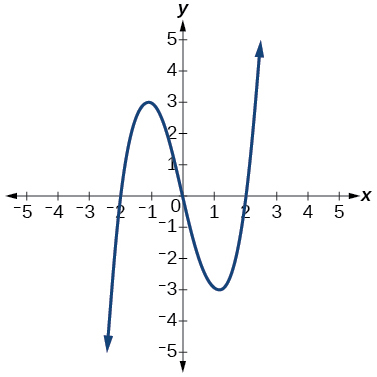function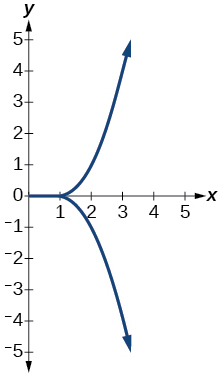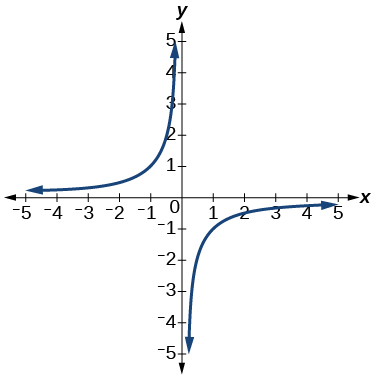function

For the following exercises, graph the functions.

$f\left(x\right)=|x+1|$

$f\left(x\right)={x}^{2}-2$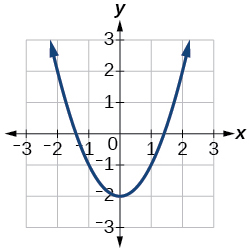For the following exercises, use [link] to approximate the values.

$f\left(2\right)$

$f\left(-2\right)$

$2$

If $\text{\hspace{0.17em}}f\left(x\right)=-2,\text{\hspace{0.17em}}$ then solve for $\text{\hspace{0.17em}}x.$

If $\text{\hspace{0.17em}}f\left(x\right)=1,\text{\hspace{0.17em}}$ then solve for $\text{\hspace{0.17em}}x.$

or

For the following exercises, use the function $\text{\hspace{0.17em}}h\left(t\right)=-16{t}^{2}+80t\text{\hspace{0.17em}}$ to find the values in simplest form.

$\frac{h\left(2\right)-h\left(1\right)}{2-1}$

$\frac{h\left(a\right)-h\left(1\right)}{a-1}$

$\frac{-64+80a-16{a}^{2}}{-1+a}=-16a+64$

## Domain and Range

For the following exercises, find the domain of each function, expressing answers using interval notation.

$f\left(x\right)=\frac{2}{3x+2}$

$f\left(x\right)=\frac{x-3}{{x}^{2}-4x-12}$

$\left(-\infty ,-2\right)\cup \left(-2,6\right)\cup \left(6,\infty \right)$

$f\left(x\right)=\frac{\sqrt{x-6}}{\sqrt{x-4}}$

Graph this piecewise function: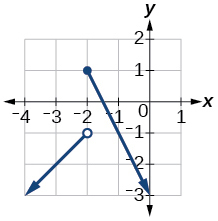## Rates of Change and Behavior of Graphs

For the following exercises, find the average rate of change of the functions from

$f\left(x\right)=4x-3$

$f\left(x\right)=10{x}^{2}+x$

$31$

$f\left(x\right)=-\frac{2}{{x}^{2}}$

For the following exercises, use the graphs to determine the intervals on which the functions are increasing, decreasing, or constant.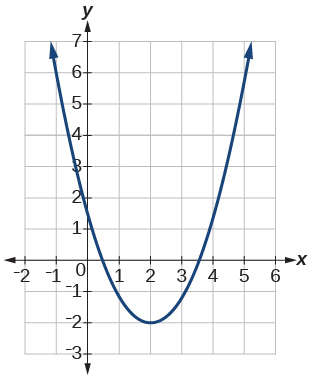increasing $\text{\hspace{0.17em}}\left(2,\infty \right);\text{\hspace{0.17em}}$ decreasing $\text{\hspace{0.17em}}\left(-\infty ,2\right)$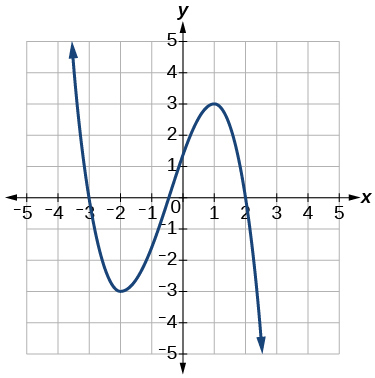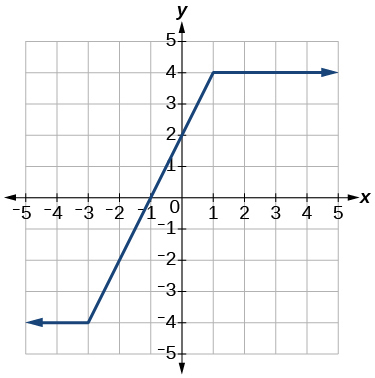increasing $\text{}\left(-3,1\right);\text{}$ constant $\text{\hspace{0.17em}}\left(-\infty ,-3\right)\cup \left(1,\infty \right)$

Find the local minimum of the function graphed in [link] .

Find the local extrema for the function graphed in [link] .

local minimum $\text{\hspace{0.17em}}\left(-2,-3\right);\text{\hspace{0.17em}}$ local maximum $\text{\hspace{0.17em}}\left(1,3\right)$

For the graph in [link] , the domain of the function is $\text{\hspace{0.17em}}\left[-3,3\right].$ The range is $\text{\hspace{0.17em}}\left[-10,10\right].\text{\hspace{0.17em}}$ Find the absolute minimum of the function on this interval.

Find the absolute maximum of the function graphed in [link] .

$\text{\hspace{0.17em}}\left(-1.8,10\right)\text{\hspace{0.17em}}$

## Composition of Functions

For the following exercises, find $\text{\hspace{0.17em}}\left(f\circ g\right)\left(x\right)\text{\hspace{0.17em}}$ and $\text{\hspace{0.17em}}\left(g\circ f\right)\left(x\right)\text{\hspace{0.17em}}$ for each pair of functions.

$f\left(x\right)=4-x,\text{\hspace{0.17em}}g\left(x\right)=-4x$

$f\left(x\right)=3x+2,\text{\hspace{0.17em}}g\left(x\right)=5-6x$

$\left(f\circ g\right)\left(x\right)=17-18x;\text{\hspace{0.17em}}\left(g\circ f\right)\left(x\right)=-7-18x$

$f\left(x\right)={x}^{2}+2x,\text{\hspace{0.17em}}g\left(x\right)=5x+1$

$\left(f\circ g\right)\left(x\right)=\sqrt{\frac{1}{x}+2};\text{\hspace{0.17em}}\left(g\circ f\right)\left(x\right)=\frac{1}{\sqrt{x+2}}$

For the following exercises, find $\text{\hspace{0.17em}}\left(f\circ g\right)\text{\hspace{0.17em}}$ and the domain for $\text{\hspace{0.17em}}\left(f\circ g\right)\left(x\right)\text{\hspace{0.17em}}$ for each pair of functions.

$\left(f\circ g\right)\left(x\right)=\frac{1}{\sqrt{x}},\text{\hspace{0.17em}}x>0$

For the following exercises, express each function $\text{\hspace{0.17em}}H\text{\hspace{0.17em}}$ as a composition of two functions $\text{\hspace{0.17em}}f\text{\hspace{0.17em}}$ and $\text{\hspace{0.17em}}g\text{\hspace{0.17em}}$ where $\text{\hspace{0.17em}}H\left(x\right)=\left(f\circ g\right)\left(x\right).$

$H\left(x\right)=\sqrt{\frac{2x-1}{3x+4}}$

sample: $\text{\hspace{0.17em}}g\left(x\right)=\frac{2x-1}{3x+4};\text{\hspace{0.17em}}f\left(x\right)=\sqrt{x}$

$H\left(x\right)=\frac{1}{{\left(3{x}^{2}-4\right)}^{-3}}$

## Transformation of Functions

For the following exercises, sketch a graph of the given function.

$f\left(x\right)={\left(x-3\right)}^{2}$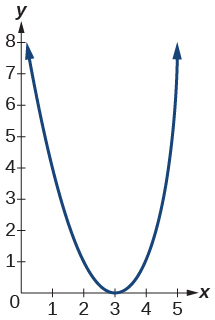$f\left(x\right)={\left(x+4\right)}^{3}$

$f\left(x\right)=\sqrt{x}+5$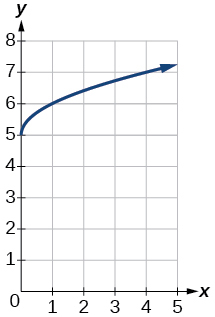$f\left(x\right)=-{x}^{3}$

$f\left(x\right)=\sqrt{-x}$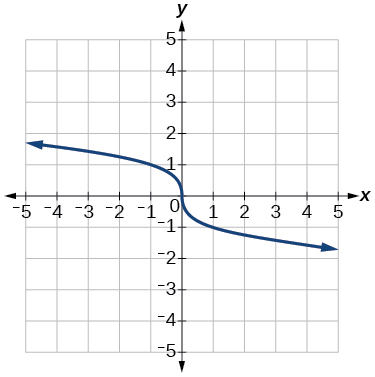$f\left(x\right)=5\sqrt{-x}-4$

$f\left(x\right)=4\left[|x-2|-6\right]$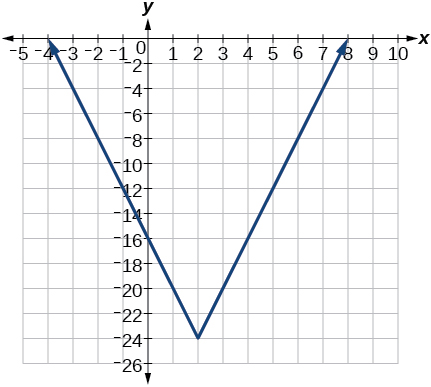$f\left(x\right)=-{\left(x+2\right)}^{2}-1$

For the following exercises, sketch the graph of the function $\text{\hspace{0.17em}}g\text{\hspace{0.17em}}$ if the graph of the function $\text{\hspace{0.17em}}f\text{\hspace{0.17em}}$ is shown in [link] .

$g\left(x\right)=f\left(x-1\right)$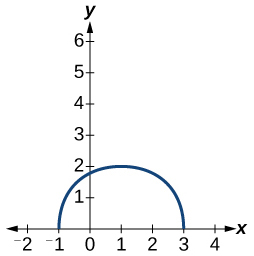$g\left(x\right)=3f\left(x\right)$

For the following exercises, write the equation for the standard function represented by each of the graphs below.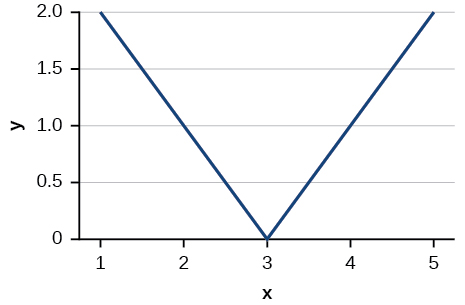$f\left(x\right)=|x-3|$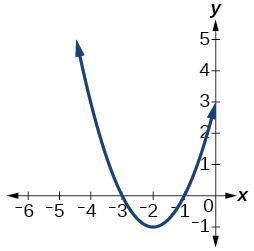For the following exercises, determine whether each function below is even, odd, or neither.

$f\left(x\right)=3{x}^{4}$

even

$g\left(x\right)=\sqrt{x}$

$h\left(x\right)=\frac{1}{x}+3x$

odd

For the following exercises, analyze the graph and determine whether the graphed function is even, odd, or neither.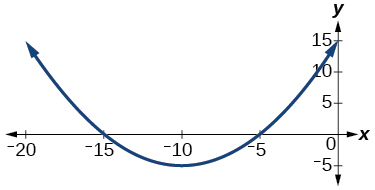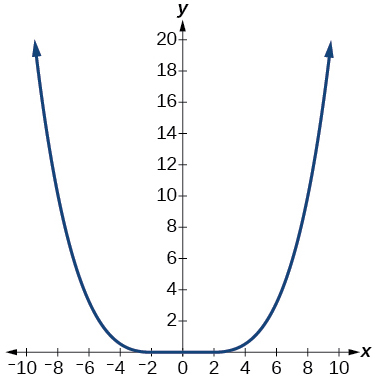even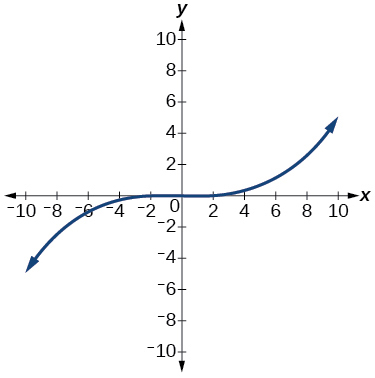## Absolute Value Functions

For the following exercises, write an equation for the transformation of $\text{\hspace{0.17em}}f\left(x\right)=|x|.$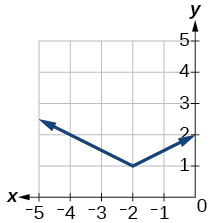$f\left(x\right)=\frac{1}{2}|x+2|+1$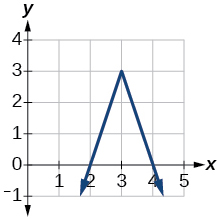$f\left(x\right)=-3|x-3|+3$

For the following exercises, graph the absolute value function.

$f\left(x\right)=|x-5|$

$f\left(x\right)=-|x-3|$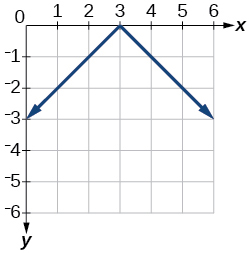$f\left(x\right)=|2x-4|$

## Inverse Functions

For the following exercises, find for each function.

$f\left(x\right)=9+10x$

$f\left(x\right)=\frac{x}{x+2}$

${f}^{-1}\left(x\right)=\frac{-2x}{x-1}$

For the following exercise, find a domain on which the function is one-to-one and non-decreasing. Write the domain in interval notation. Then find the inverse of restricted to that domain.

$f\left(x\right)={x}^{2}+1$

Given $f\left(x\right)={x}^{3}-5$ and $g\left(x\right)=\sqrt{x+5}:$

1. Find and $g\left(f\left(x\right)\right).$
2. What does the answer tell us about the relationship between $f\left(x\right)$ and $g\left(x\right)?$
1. and $g\left(f\left(x\right)\right)=x.$
2. This tells us that $f$ and $g$ are inverse functions

For the following exercises, use a graphing utility to determine whether each function is one-to-one.

$f\left(x\right)=\frac{1}{x}$

The function is one-to-one.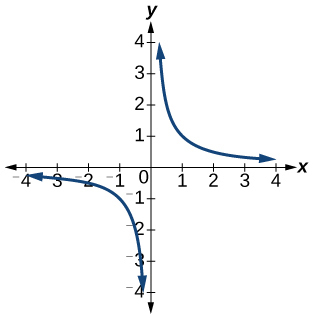$f\left(x\right)=-3{x}^{2}+x$

The function is not one-to-one.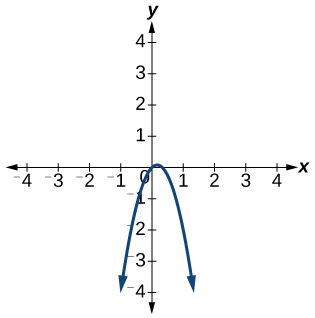If $f\left(5\right)=2,$ find ${f}^{-1}\left(2\right).$

$5$

If $f\left(1\right)=4,$ find ${f}^{-1}\left(4\right).$

## Practice test

For the following exercises, determine whether each of the following relations is a function.

$y=2x+8$

The relation is a function.

$\left\{\left(2,1\right),\left(3,2\right),\left(-1,1\right),\left(0,-2\right)\right\}$

For the following exercises, evaluate the function $\text{\hspace{0.17em}}f\left(x\right)=-3{x}^{2}+2x\text{\hspace{0.17em}}$ at the given input.

$f\left(-2\right)$

−16

$\text{\hspace{0.17em}}f\left(a\right)\text{\hspace{0.17em}}$

Show that the function $\text{\hspace{0.17em}}f\left(x\right)=-2{\left(x-1\right)}^{2}+3\text{\hspace{0.17em}}$ is not one-to-one.

The graph is a parabola and the graph fails the horizontal line test.

Write the domain of the function $\text{\hspace{0.17em}}f\left(x\right)=\sqrt{3-x}\text{\hspace{0.17em}}$ in interval notation.

Given $\text{\hspace{0.17em}}f\left(x\right)=2{x}^{2}-5x,\text{\hspace{0.17em}}$ find $f\left(a+1\right)-f\left(1\right)\text{\hspace{0.17em}}$ in simplest form.

$2{a}^{2}-a$

Graph the function

Find the average rate of change of the function $\text{\hspace{0.17em}}f\left(x\right)=3-2{x}^{2}+x\text{\hspace{0.17em}}$ by finding $\text{\hspace{0.17em}}\frac{f\left(b\right)-f\left(a\right)}{b-a}\text{\hspace{0.17em}}$ in simplest form.

$-2\left(a+b\right)+1$

For the following exercises, use the functions to find the composite functions.

$\left(g\circ f\right)\left(x\right)$

$\left(g\circ f\right)\left(1\right)$

$\sqrt{2}$

Express $\text{\hspace{0.17em}}H\left(x\right)=\sqrt{5{x}^{2}-3x}\text{\hspace{0.17em}}$ as a composition of two functions, $\text{\hspace{0.17em}}f\text{\hspace{0.17em}}$ and $\text{\hspace{0.17em}}g,\text{\hspace{0.17em}}$ where $\text{\hspace{0.17em}}\left(f\circ g\right)\left(x\right)=H\left(x\right).$

For the following exercises, graph the functions by translating, stretching, and/or compressing a toolkit function.

$f\left(x\right)=\sqrt{x+6}-1$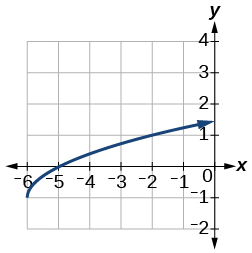$f\left(x\right)=\frac{1}{x+2}-1$

For the following exercises, determine whether the functions are even, odd, or neither.

$f\left(x\right)=-\frac{5}{{x}^{2}}+9{x}^{6}$

$\text{even}$

$f\left(x\right)=-\frac{5}{{x}^{3}}+9{x}^{5}$

$f\left(x\right)=\frac{1}{x}$

$\text{odd}$

Graph the absolute value function $\text{\hspace{0.17em}}f\left(x\right)=-2|x-1|+3.$

For the following exercises, find the inverse of the function.

$f\left(x\right)=3x-5$

${f}^{-1}\left(x\right)=\frac{x+5}{3}$

$f\left(x\right)=\frac{4}{x+7}$

For the following exercises, use the graph of $\text{\hspace{0.17em}}g\text{\hspace{0.17em}}$ shown in [link] .

On what intervals is the function increasing?

On what intervals is the function decreasing?

Approximate the local minimum of the function. Express the answer as an ordered pair.

$\left(1.1,-0.9\right)$

Approximate the local maximum of the function. Express the answer as an ordered pair.

For the following exercises, use the graph of the piecewise function shown in [link] .

Find $\text{\hspace{0.17em}}f\left(2\right).$

$f\left(2\right)=2$

Find $\text{\hspace{0.17em}}f\left(-2\right).$

Write an equation for the piecewise function.

$f\left(x\right)=\left\{\begin{array}{c}|x|\text{\hspace{0.17em}}\text{\hspace{0.17em}}\text{\hspace{0.17em}}\text{if}\text{\hspace{0.17em}}\text{\hspace{0.17em}}x\le 2\\ 3\text{\hspace{0.17em}}\text{\hspace{0.17em}}\text{\hspace{0.17em}}\text{\hspace{0.17em}}\text{\hspace{0.17em}}\text{if}\text{\hspace{0.17em}}\text{\hspace{0.17em}}x>2\end{array}$

For the following exercises, use the values listed in [link] .

$x$ $F\left(x\right)$
0 1
1 3
2 5
3 7
4 9
5 11
6 13
7 15
8 17

Find $\text{\hspace{0.17em}}F\left(6\right).$

Solve the equation $\text{\hspace{0.17em}}F\left(x\right)=5.$

$x=2$

Is the graph increasing or decreasing on its domain?

Is the function represented by the graph one-to-one?

yes

Find $\text{\hspace{0.17em}}{F}^{-1}\left(15\right).$

Given $\text{\hspace{0.17em}}f\left(x\right)=-2x+11,\text{\hspace{0.17em}}$ find $\text{\hspace{0.17em}}{f}^{-1}\left(x\right).$

${f}^{-1}\left(x\right)=-\frac{x-11}{2}$

sinx sin2x is linearly dependent
what is a reciprocal
The reciprocal of a number is 1 divided by a number. eg the reciprocal of 10 is 1/10 which is 0.1
Shemmy
Reciprocal is a pair of numbers that, when multiplied together, equal to 1. Example; the reciprocal of 3 is ⅓, because 3 multiplied by ⅓ is equal to 1
Jeza
each term in a sequence below is five times the previous term what is the eighth term in the sequence
I don't understand how radicals works pls
How look for the general solution of a trig function
stock therom F=(x2+y2) i-2xy J jaha x=a y=o y=b
sinx sin2x is linearly dependent
cr
root under 3-root under 2 by 5 y square
The sum of the first n terms of a certain series is 2^n-1, Show that , this series is Geometric and Find the formula of the n^th
cosA\1+sinA=secA-tanA
Wrong question
why two x + seven is equal to nineteen.
The numbers cannot be combined with the x
Othman
2x + 7 =19
humberto
2x +7=19. 2x=19 - 7 2x=12 x=6
Yvonne
because x is 6
SAIDI
what is the best practice that will address the issue on this topic? anyone who can help me. i'm working on my action research.
simplify each radical by removing as many factors as possible (a) √75
how is infinity bidder from undefined?
what is the value of x in 4x-2+3
give the complete question
Shanky
4x=3-2 4x=1 x=1+4 x=5 5x
Olaiya
hi can you give another equation I'd like to solve it
Daniel
what is the value of x in 4x-2+3
Olaiya
if 4x-2+3 = 0 then 4x = 2-3 4x = -1 x = -(1÷4) is the answer.
Jacob
4x-2+3 4x=-3+2 4×=-1 4×/4=-1/4
LUTHO
then x=-1/4
LUTHO
4x-2+3 4x=-3+2 4x=-1 4x÷4=-1÷4 x=-1÷4
LUTHO
A research student is working with a culture of bacteria that doubles in size every twenty minutes. The initial population count was  1350  bacteria. Rounding to five significant digits, write an exponential equation representing this situation. To the nearest whole number, what is the population size after  3  hours?
f(x)= 1350. 2^(t/20); where t is in hours.
MerkebByByBy Saylor FoundationBy Dindin SecretoBy Edgar DelgadoBy Ann SchlosserBy Jonathan LongBy Jams KaloBy RhodesBy John GabrieliBy Saylor FoundationBy OpenStax